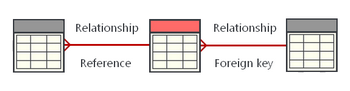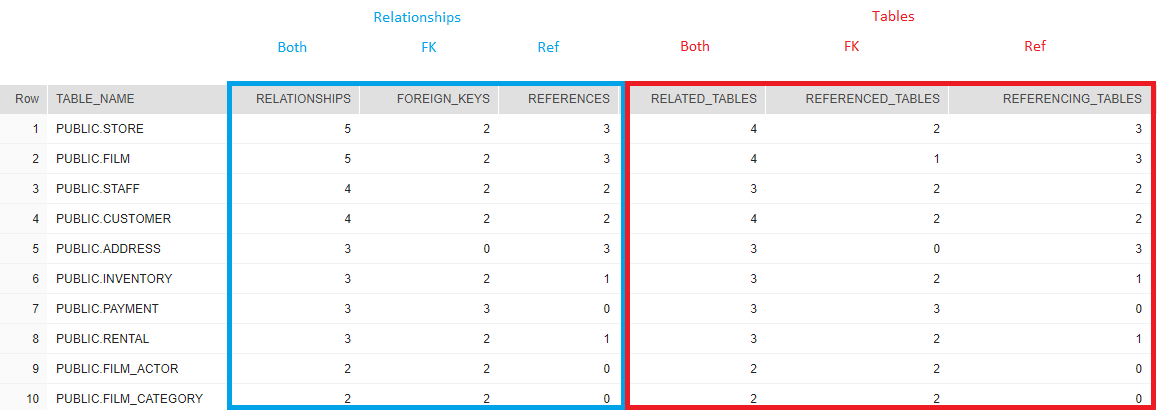## List tables with most relationships in Snowflake

Article for:

Query below returns relationships statistics (foreign keys and references from other tables).## Query

``````select relations.table_name as table_name,
count(relations.table_name) as relationships,
count(relations.referenced_tables) as foreign_keys,
count(relations.referencing_tables) as references,
count(distinct related_table) as related_tables,
count(distinct relations.referenced_tables) as referenced_tables,
count(distinct relations.referencing_tables) as referencing_tables
from(
select pk_tco.table_schema ||'.' || pk_tco.table_name as table_name,
fk_tco.table_schema ||'.'|| fk_tco.table_name as related_table,
fk_tco.table_name as referencing_tables,
null as referenced_tables
from information_schema.referential_constraints rco
join information_schema.table_constraints fk_tco
on rco.constraint_name = fk_tco.constraint_name
and rco.constraint_schema = fk_tco.table_schema
join information_schema.table_constraints pk_tco
on rco.unique_constraint_name = pk_tco.constraint_name
and rco.unique_constraint_schema = pk_tco.table_schema
union all
select fk_tco.table_schema ||'.'|| fk_tco.table_name as table_name,
pk_tco.table_schema ||'.'|| pk_tco.table_name as related_table,
null as referencing_tables,
pk_tco.table_name as referenced_tables
from information_schema.referential_constraints rco
join information_schema.table_constraints fk_tco
on rco.constraint_name = fk_tco.constraint_name
and rco.constraint_schema = fk_tco.table_schema
join information_schema.table_constraints pk_tco
on rco.unique_constraint_name = pk_tco.constraint_name
and rco.unique_constraint_schema = pk_tco.table_schema
) relations
group by table_name
order by relationships desc;
``````

## Columns

• table_name - name of table preceded by schema name
• relationships - number of relationships
• foreign_keys - number of FKs
• references - number of FK references from other tables
• related_tables - number of different related tables (table is counted only once regardless of relationship type/direction )
• referenced_tables - number of different tables referenced with FKs (number of FKs and number of referenced tables can be different, because multiple FK's in one table can refer to single table)
• referencing_tables - number of different tables referencing this table with foreign keys

## Rows

• One row represents one table in a database
• Scope of rows: all tables which have at least one relationship
• Ordered by number of relationships from the ones with the most

## Sample results